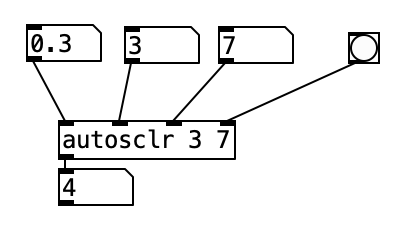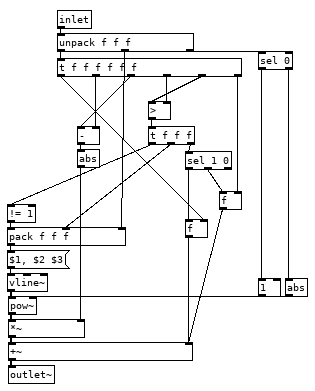• ### ways of exponentiation ( / range mapping)

Hi,

I need to be able to convert a value within a given range to an exponential value within the same range. I made an abstraction "autoscale" which remap the highest and lowest received values to a given range.

If incoming is 0.3 and the lowest value so far been 0.2, the highest so far has been 1.2, and the range is set to 3 and 7 the output will be 4.

I would like to be able modify the output value to an exponential or logarithmic curve where you can set the curve steepness.

What options would there be to solve this? Could I have a function within an expression object? Or use an array? Please show me.

Once I got that going I need to be able to set the curve so that a given value will end up as the exact half of the scaled range. Using the values in the example above incoming value 0.3 should then output 5 after the exponential curve has been set.autosclr.pd

Cheers!

• Posts 8 | Views 1624
• @cfry Way back when I had no real clue what I was doing I did this programmable curve Should give you a starting point. I did not understand what [vline~] did back then, it would simplify things and there is no reason for that big [expr] but I had not quite figured out order of operations yet and that is the only way I could make it work.

Edit; I went ahead and fixed it up a bit. Here it is without the input timing stuff. Just send it a message of target value, duration and a power 0.5 for log, 2 for expo and anything between or further to the extreme for steeper or gentler curves. [1 1000 2( would give an expo curve ramping too 1 over 1 second. Remembered that I did not use [vline~] because still need to time the curve value and scaling.
curver~.pdEdit, fixed some silliness, probably still some more in there.

I would like to be able modify the output value to an exponential or logarithmic curve where you can set the curve steepness.

[pow] needs an incoming range between 0 and 1 for this.

input
map from input range to 0 till 1
[pow]
map from 0 till 1 to output range
output

Or use an array?

Would be similar:
draw an array with a transfer-function

input
map to x-size of array
map from y-range
output

• Thank you for the input @oid @lacuna, [pow] was all that I needed but curver is a keeper.

• More generally, this is range mapping, absolutely central to any type of interactive work. I usually teach it like this:

You have a base value. This could be one end of the range, or the center of the range.

You have some input data -- could be LFO, envelope, or external data.

Normalize the input -- if the base value is the end of the output range, then your normalized input would be 0 .. 1. If it's the center, then the normalized input is -1 .. +1.

Then linear mapping is `base + ((range_end - base) * normalized_value)`. I write it in this order because... then...

Exponential ("log") mapping only promotes the operators up one level: `base * ((range_end / base) ** normalized_value)` where `**` is "pow" in Pd. (Note that range_end / base needs to be positive and nonzero.)

You can translate from any input range to any output range this way.

For variable-curvature mapping, I translated the formula from SuperCollider in my abstraction library, the [lincurve] and [lincurve~] objects.

hjh

• @ddw_music thank you for this excellent input. I would actually prefer to just use the equation inside an expression object, feels it should be more intuitive.

• @cfry said:

I would actually prefer to just use the equation inside an expression object

Without testing, I believe the SC lincurve formula could be written into expr as:

``````if(abs(\$f6) < 0.001, (\$f1 - \$f2) / (\$f3 - \$f2) * (\$f5 - \$f4) + \$f4, \$f4 + ((\$f5 - \$f4) / (1.0 - exp(\$f6))) - (((\$f5 - \$f4) / (1.0 - exp(\$f6))) * pow(exp(\$f6), (\$f1 - \$f2) / (\$f3 - \$f2))))
``````

Where:

\$f1 = input
\$f2 = input lower bound
\$f3 = input upper bound
\$f4 = output lower bound
\$f5 = output upper bound
\$f6 = curve

YMMV.

hjh

• the easiest way is to break it down into three parts.

1.) map it down to range 0-1,
2.) for log, do log((\$f1)* (\$f2-1)+1)/log(\$f2)
3.) then map it back to its original range.

for exp, use (exp(\$f1*log(\$f2))-1)/(\$f2-1),

to turn the curve upside down, invert the range to 1-0 and so on.

Posts 8 | Views 1624
Internal error.

Oops! Looks like something went wrong!